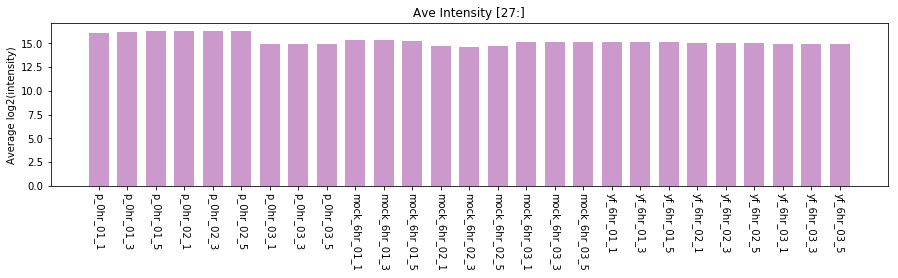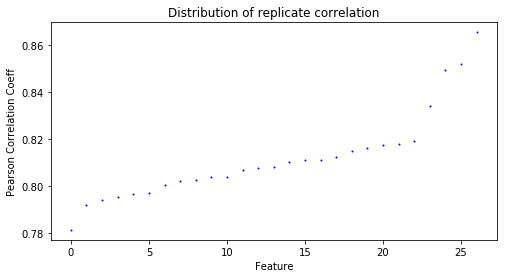# Averaging technical replicates¶

Each biological sample was run in triplicates. Taking geometric mean of technical replicates.

Example data are from Li et al. (2013) Predicting network activity from high throughput metabolomics. PLoS computational biology 9.7 (2013): e1003123.

In :
import os
import numpy as np
import pandas as pd
import matplotlib.pyplot as plt

%matplotlib inline

INDIR = "../input_data/"
datafile = "modc_ae_2012.txt"

Out:
mz time mz.min mz.max p_0hr_01_1 p_0hr_01_3 p_0hr_01_5 p_0hr_02_1 p_0hr_02_3 p_0hr_02_5 ... mock_6hr_03_5 yf_6hr_01_1 yf_6hr_01_3 yf_6hr_01_5 yf_6hr_02_1 yf_6hr_02_3 yf_6hr_02_5 yf_6hr_03_1 yf_6hr_03_3 yf_6hr_03_5
0 85.02783 59.68820 85.02783 85.02783 15.5810 16.0425 15.3953 15.4143 15.7364 15.5532 ... 17.5140 17.1734 16.9813 16.6582 16.8376 16.8148 17.2885 16.5967 16.4734 16.6048
1 85.04717 124.75120 85.04709 85.04739 14.4754 14.2709 14.3696 14.7142 14.8291 14.9959 ... 16.6485 16.6961 16.3596 17.0661 15.2321 15.1615 16.5655 16.4316 16.1668 16.2193
2 85.06532 68.66651 85.06517 85.06547 14.4223 15.0515 15.1241 13.5224 16.7480 14.4095 ... 9.7859 14.0113 15.8681 13.3699 9.9658 15.5703 16.5537 11.9470 10.3181 10.1656
3 85.10073 16.48022 85.10050 85.10078 14.5249 13.2573 13.5206 12.9044 11.4185 12.9223 ... 13.3513 13.2977 13.1140 12.5569 12.9582 12.9960 13.0290 12.4184 13.0218 13.4085
4 86.05951 67.78485 86.05949 86.05980 10.6674 17.4235 17.4510 17.0197 17.0402 16.9958 ... 18.5019 17.7391 17.9262 17.6089 17.5802 17.6529 17.7841 17.3077 10.7589 17.3895

5 rows × 31 columns

This is a dataset of human monocyte-derived dendritic cells (moDC). Three experimental groups are baseline control, mock infection at 6 hours, YFV infection at 6 hours. Each group contains three biological replicates. Each biological replicate was analyzed by LC-MS in three technical replicates. This above table was extracted using apLCMS.

## Checking data quality by¶

• average ion intensity in each sample
• correlation coefficient between technical replicates
In :
new_header = [x for x in data]

# if needed to transform to log2, shift positive 1 to avoid log2(0)
# new_data = np.log2(mydata[ new_header[4:] ] + 1)

mean_TIC = new_data.mean(0)
# plot bars for first N columns, useful if large number of samples
N = 27
ind = np.arange(N)
plt.figure(figsize=(15,3))
plt.bar(ind, mean_TIC[:N], width=0.7, color="purple", alpha=0.4)
plt.ylabel("Average log2(intensity)")
plt.title("Ave Intensity [%d:]" %N)
plt.xticks(ind, new_data.columns[:N], rotation=-90)
plt.show()

# one should be alarmed if irregularity is spotted. Replicate of low intensity may be subject of removal.
# Alternatively, pandas dataframe has built-in plotting too (also via matplotlib)In :
# We are making a new dictionary to map samples to replicates

def remove_tail(x):
return("_".join(x.split("_")[:-1]))

samples = [remove_tail(x) for x in new_header[4:]]
print("After remove_tail: ", samples[:6])

samples = set(samples)
samDict = {}
k = remove_tail(x)
if samDict.has_key(k):
samDict[k].append(x)
else:
samDict[k] = [x,]

print("Example sample dictionary:")
print(samDict.items()[:3])

('After remove_tail: ', ['p_0hr_01', 'p_0hr_01', 'p_0hr_01', 'p_0hr_02', 'p_0hr_02', 'p_0hr_02'])
Example sample dictionary:
[('yf_6hr_02', ['yf_6hr_02_1', 'yf_6hr_02_3', 'yf_6hr_02_5']), ('yf_6hr_03', ['yf_6hr_03_1', 'yf_6hr_03_3', 'yf_6hr_03_5']), ('yf_6hr_01', ['yf_6hr_01_1', 'yf_6hr_01_3', 'yf_6hr_01_5'])]

In :
# Pearson correlation btw technical replicates
# plot first before deciding CUTOFF value

def get_flat_corr(CorrDataFrame):
return [CorrDataFrame.values[0, 1], CorrDataFrame.values[0, 2], CorrDataFrame.values[1, 2]]

all_Pearson_coefficients = []
for k,v in samDict.items():
if len(v) == 3:  # only do if three replicates
Pr = new_data[v].corr()
all_Pearson_coefficients.append((v, Pr))

all_corr_values = []
for X in all_Pearson_coefficients:
all_corr_values += get_flat_corr(X)

# This is useful where sample nubmer is large
all_corr_values.sort()
plt.figure(figsize=(8,4))
plt.plot(range(len(all_corr_values)), all_corr_values, 'bo', markersize=1)
plt.title("Distribution of replicate correlation")
plt.xlabel("Feature")
plt.ylabel("Pearson Correlation Coeff")
plt.show()In :
# This block is to remove bad replicates.
#
# CUTOFF is based on plot above; this is usually dragged down by too many zeros
# This does nothing because the worst is 0.78 in above figure
corr_CUTOFF = 0.7

# Deterine which replicate to remove
# assuming three replicates here,
# return list of bad replicates
names = [x for x in CorrDataFrame]
good = []
if CorrDataFrame.values[0, 1] > corr_CUTOFF:
good += [names, names]

if CorrDataFrame.values[0, 2] > corr_CUTOFF:
good += [names, names]

if CorrDataFrame.values[1, 2] > corr_CUTOFF:
good += [names, names]

return [x for x in names if x not in good]

for X in all_Pearson_coefficients:
# uncomment below to see the whole corr matrix
#print(Pr)

# In some dataset, many Qstd samples have the same name. Skipping them for now.
# Alternative method can use stats.pearsonr and itertools.combinations


## Averaging technical replicates¶

Since data are already in log2, myave function is actually taking geometric means.

In :
def myave(L):
new = [x for x in L if x >1]
if new:
return np.mean(new)
else:
return 0

# update samDict
# this is used when bad replicates need to be excluded
# black_list = set(low_intensity_replicates + bad_replicates)
#
black_list = []
samDict2 = {}
for k,v in samDict.items():
new = [x for x in v if x not in black_list]
if new:
# We may want to keep NIST and Q-std samples for other reasons including downstream QC
if 'nist' not in k and 'q3' not in k:
samDict2[k] = new

# Write ave_log2_ data
use_samples = samDict2.keys()
use_samples.sort()
s = 'mz\tretention_time\t' + '\t'.join(use_samples) + '\n'

# mydata contains mz, this set of data is already in log2
print(data.shape)
num_features = data.shape

print("use_samples", use_samples[:3])
print(samDict2[use_samples])

# mapper is faster than DataFrame lookup
new_data_header = [x for x in new_data]
mapper = []
for x in use_samples:

for ii in range(num_features):
mz, rtime = data.values[ii, :2]
d = new_data.values[ii, :]
ave_data = [myave( [d[ii] for ii in x] ) for x in mapper]
s += '\t'.join( [str(mz), str(int(rtime)), ] + [str(round(x,2)) for x in ave_data] ) + '\n'

new_file = "ave_log2_" + datafile
with open(new_file, 'w') as file:
file.write(s)

(7995, 31)
('use_samples', ['mock_6hr_01', 'mock_6hr_02', 'mock_6hr_03'])
['mock_6hr_03_1', 'mock_6hr_03_3', 'mock_6hr_03_5']


## Summary¶

The above code illustrates simple QC and averaging replicates.

Not covered here, but filtering, normalization and imputation is often needed. Missing values can be problematic for some statistical methods, including linear regression. Data should be filtered to have fewer missing values. The missing values can be imputed by replacement of minimal values of a feature row (i.e. minimal detected values in the same feature). Other imputation methods are available too. Imputation may be performed prior to normalization, as the normalization method may be sensitive to missing data.